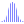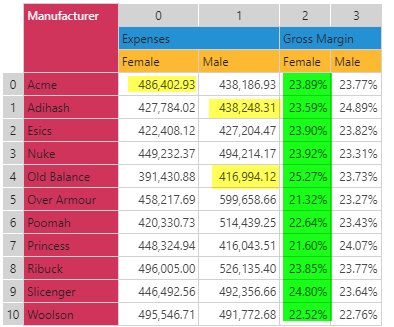#Rank (PQL)

Finds the rank position of a number in a list of numbers.

• Returned Output: Numeric
• Library: PQL \ Common \ Statistical
• Version: 2018.00.000
• Compatibility:
• Can be combined with and other PQL function throughout the application.
• It CANNOT be used with MDX or VBA functions. But it can be used on MDX-based content in other parts of the application.

#### Syntax

Rank( <Numeric> , )

* Click on the function's arguments above for more details on the input values.

• The list of numbers can be typed in as a comma delimited list or inserted via an array.
• The rank function finds the position of the supplied target value in the value list, based on a descending sort of the numbers in the list.
##### Different Function types
• This function is like the Excel RANK function - calculating the rank of a number from a list of items.
• Also see Percentile Rank.

#### Examples

This example finds the rank of the value 4 in the list of values 3 to 6 (returning 3). The dataset function returns a list of numbers.

rank(4, dataset(3,4,5,6))This example, using the grid above, would find the rank of a number across all the values in column 2 (numbering is zero-based). The DataSetColumn function returns an array (list) of values from column 3 in the grid above.

rank(0.22, dataSetColumn(2))

So it would rank of 22% in a list of all items 23.89%, 23.59% etc will return 9.